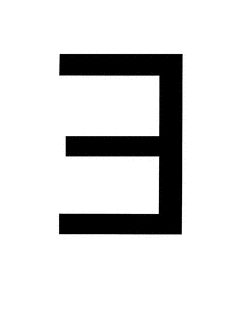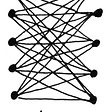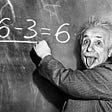# Chebyshev’s Inequality in Statistics — Explained

This article could be considered as an introduction for beginners or a quick refresher for experienced.

# What is Chebyshev’s Inequality?

Chebyshev’s theorem is used to determine the proportion of events you would expect to find within a certain number of standard deviations from the mean.

For normal distributions, about 68% of results will fall between +1 and -1 standard deviations from the mean. About 95% will fall between +2 and -2 standard deviations.

But what if our random variable is not in Normal distribution, and when they are not in Normal Distribution how do we find the number of events that lie under a certain number of standard deviations from the mean. It is the reason we use Chebyshev’s Inequality.

For a population or sample, the proportion of observations is no less than

(1 — (1 / k² ))

Here the value of K is the number of Standard Deviations from the mean. For example, let’s take the Second Standard deviation.

Then our k = 2

=> (1–1/4) => 3/4 => 0.75

This value of 0.75 means that more than 75% of events will lie under the second Standard Deviation.

# Conclusion

There is one thing that you should keep in mind before using Chebyshev’s Inequality that is “ The random variable must not be in Normal distribution”. If the variable is not in Normal distribution you can use Chebyshev’s Inequality and find greater than how many events like under a certain number of standard deviations from the mean.

Don’t forget to leave your responses.

Everyone stay tuned! To get my stories in your mailbox kindly subscribe to my newsletter.

Thank you for reading! Do not forget to give your claps and to share your responses and share it with a friend!

The original blog on Fittechie

--

--

--

## More from Adith - The Data Guy

Love podcasts or audiobooks? Learn on the go with our new app.

## There Exists An Elegant, Lovely, and Inspiring School Mathematics.## Tensor Products — Linear Algebra for QC## Linear regression for 5-year-olds## The Exception That Proves the Rule## From computational electromagnetics to financial derivatives: The method of finite differences## The most basic mathematical concepts to start learning the Theory of Relativity and Spacetime## Genetic Algorithm (GA) series## Linear Regression : A linear quest for relationship## Linear Regression From Scratch- 3 Methods## The Math Behind K-Means Clustering